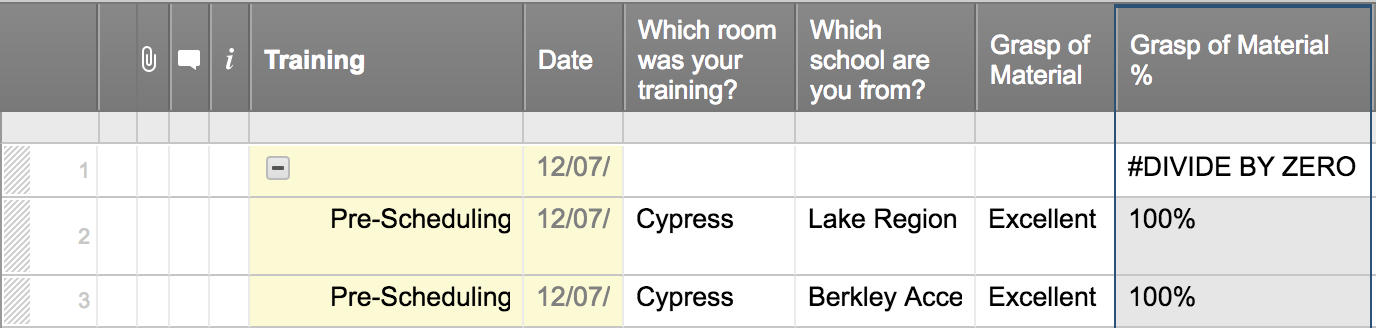#### Welcome to the Smartsheet Forum Archives

The posts in this forum are no longer monitored for accuracy and their content may no longer be current. If there's a discussion here that interests you and you'd like to find (or create) a more current version, please Visit the Current Forums.

# Formula puts data left-justified instead of right-justified

I have the following simple formula:

=IF([Grasp of Material]3 = "Excellent", "100%", IF([Grasp of Material]3 = "Good", "80%", IF([Grasp of Material]3 = "Fair", "60%", IF([Grasp of Material]3 = "Poor", "40%", IF([Grasp of Material]3 = "N/A", "N/A")))))

The formula puts the 100%, 80%, etc. as left-justified. When I try to =AVG(CHILDREN()) for that column, it produces #DIVIDE BY ZERO. If I manually enter the 100% though, this data is right-justified and the =AVG(CHILDREN()) works. How do I get the =AVG(CHILDREN()) to work in coordination with the formula?

Thanks in advance for any help!Tags:

• When your formula returns "60%", it is returning Text value. Text values are normally left-justified. If your formula returns 0.6, it will be a Number. Numbers are normally right-justified.

If the column (or cell) is formatted as a % (from the tool bar), then 0.6 is shown as 60%, but the value underneath is still 0.6. 0 to 1 is 0 to 100%

Change your formula to use Numbers

=IF([Grasp of Material]3 = "Excellent", 1, IF([Grasp of Material]3 = "Good", 0.8, IF([Grasp of Material]3 = "Fair", "0.6, IF([Grasp of Material]3 = "Poor",0.4, IF([Grasp of Material]3 = "N/A", "N/A")))))

Note that "N/A" is not a 0 for purposes of the AVG calculation so if there are 4 values of 100% and 6 values of N/A, the average is 100%. That can be accounted for too, if that is not your intent.

Lastly, if you use "60" instead of "60%", you could 'cast' the 60 Text to a number using VALUE(), but that is sometimes cumbersome and would be in your example.

Craig

• That is exactly what I needed. Thank you, Craig!

Halie

This discussion has been closed.